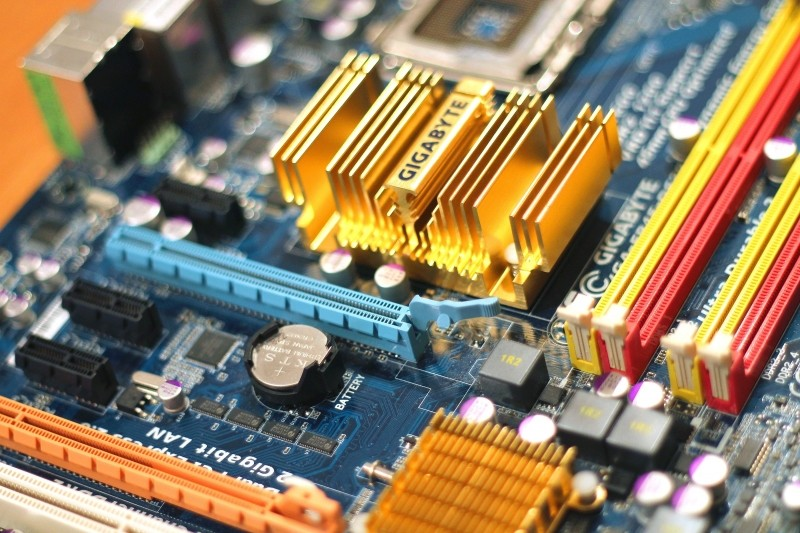# 電腦常見單位: bit、bps、byte、octet、word、KB、KiB…

## 位元/秒 (bps)

1 Kbps = 103 bit/s = 每秒傳輸 1,000 bit
1 Mbps = 106 bit/s = 每秒傳輸 1,000,000 bit
1 Gbps = 109 bit/s = 每秒傳輸 1,000,000,000 bit

## Octet

Octet，為 8 = 23 個位元。

(ex: [RFC5234], Appendix B.1)

[註1]:

## KB、KiB、MB、MiB…

(ex: 你買了 8G 隨身碟，以為是 8 * 1024 MB，卻發現比想像中的小很多 😭)

KB (Kilobyte) = 1000 byte = 103 byte = 千位元組
MB (Megabyte) = 1000 KB = 106 byte = 百萬位元組
GB (Gigabyte) = 1000 MB = 109 byte = 十億位元組
TB (Terabyte) = 1000 GB = 1012 byte = 兆位元組
PB (Petabyte) = 1000 TB = 1015 byte = 千兆位元組
EB (Exabyte) = 1000 PB = 1018 byte = 艾 (艾可薩) 位元組

KiB (kibibyte) = 1024 byte = 210 byte
MiB (Mebibyte) = 1024 KB = 220 byte
GiB (Gibibyte) = 1024 MB = 230 byte
TiB (Tebibyte) = 1024 GB = 240 byte
PiB (Pebibyte) = 1024 TB = 250 byte
EiB (Exbibyte) = 1024 PB = 260 byte### 位元速率

ex:

KB/s = kilobyte per second = 103 byte/s

KiB/s = kibibyte per second = 210 byte/s